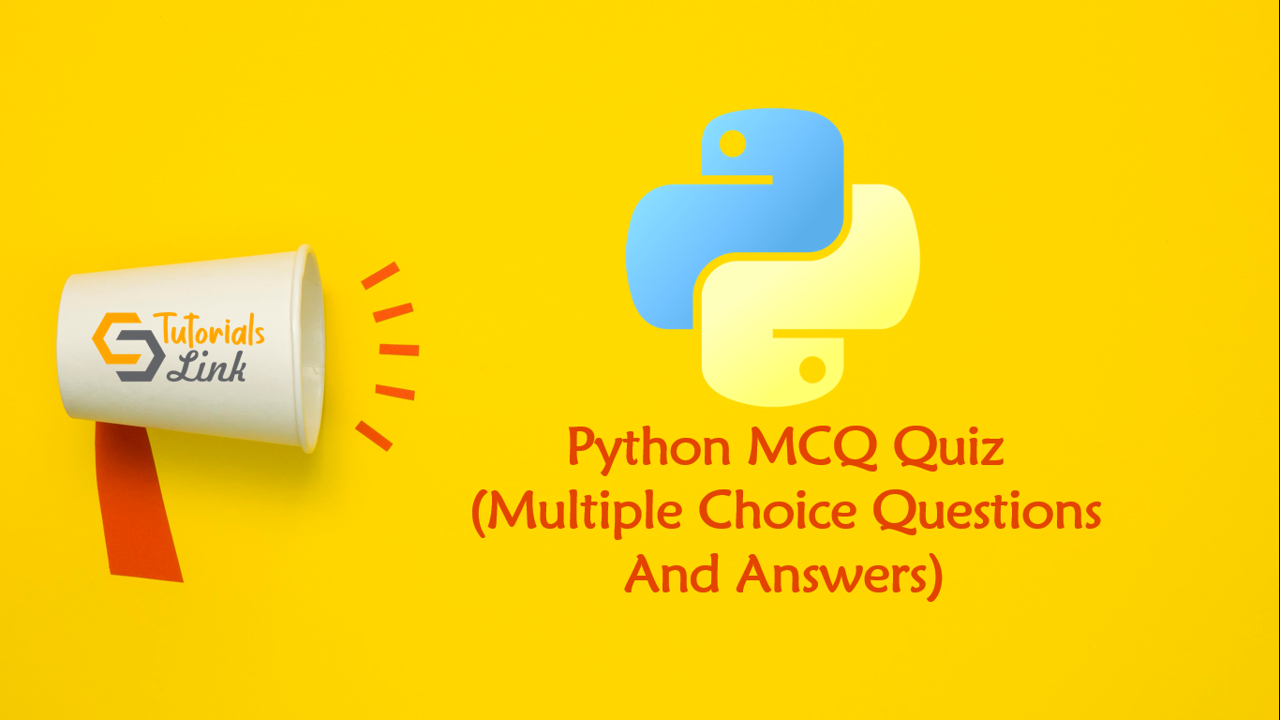Loading, please wait...# Python Multiple Choice Questions And Answers

Search here for MCQs

Are you preparing for the next job interviews? If yes, trust me this post will help you also we'll suggest you check out a big collection for Programming Full Forms that may help you in your interview:

## 11. What will be the output of the following Python list comprehension?

[j for i in range(2,8) for j in range(i*2, 50, i)]

The list comprehension shown above returns a list of non-prime numbers up to 50. The logic behind this is that the square root of 50 is almost equal to 7. Hence all the multiples of 2-7 are not prime in this range.

• A list of prime numbers up to 50
• A list of numbers divisible by 2, up to 50
• A list of non prime numbers, up to 50
• Error

## 12. The function pow(x,y,z) is evaluated as:

The built-in function pow() can accept two or three arguments. When it takes in two arguments, they are evaluated as x**y. When it takes in three arguments, they are evaluated as (x**y)%z.
• (x**y)**z
• (x**y) / z
• (x**y) % z
• (x**y)*z

## 13. What will be the output of the following Python function?

``min(max(False,-3,-4), 2,7)``

The function max() is being used to find the maximum value from among -3, -4 and false. Since false amounts to the value zero, hence we are left with min(0, 2, 7) Hence the output is 0 (false).

• 2
• False
• -4

## 14. What will be the output of the following Python code?

``````my_tuple = (1, 2, 3, 4)
my_tuple.append( (5, 6, 7) )
print len(my_tuple)``````

Tuples are immutable and don’t have an append method. An exception is thrown in this case.

• 1
• 2
• Error

## 15. What will be the output of the following Python code?

l=[2, 3, [4, 5]] l2=l.copy() l2=88 l l2 The code shown above depicts deep copy. In deep copy, the base address of the objects is not copied. Hence the modification done on one list does not affect the other list.
• [88, 2, 3, [4, 5]] [88, 2, 3, [4, 5]]
• [2, 3, [4, 5]] [88, 2, 3, [4, 5]
• [88, 2, 3, [4, 5]] [2, 3, [4, 5]]
• [2, 3, [4, 5]] [2, 3, [4, 5]]